# RD Sharma Solutions for Class 11 Chapter 23 - The Straight Lines Exercise 23.7

In this section, we shall study about one of the different forms of the equation of a straight line i.e., normal form or perpendicular form of a line, with examples for concepts to be clear enough for students to understand. Students can use the solutions pdf in order to know a better way of solving problems easily. The solutions are designed by experts at BYJU’S, keeping in mind the understanding ability of students. For better conceptual knowledge, students can make use of RD Sharma Class 11 Maths Solutions, which is available in the pdf format. Students can download the pdf from the links given below.

## Download the pdf of RD Sharma Solutions for Class 11 Maths Exercise 23.7 Chapter 23 – The Straight Lines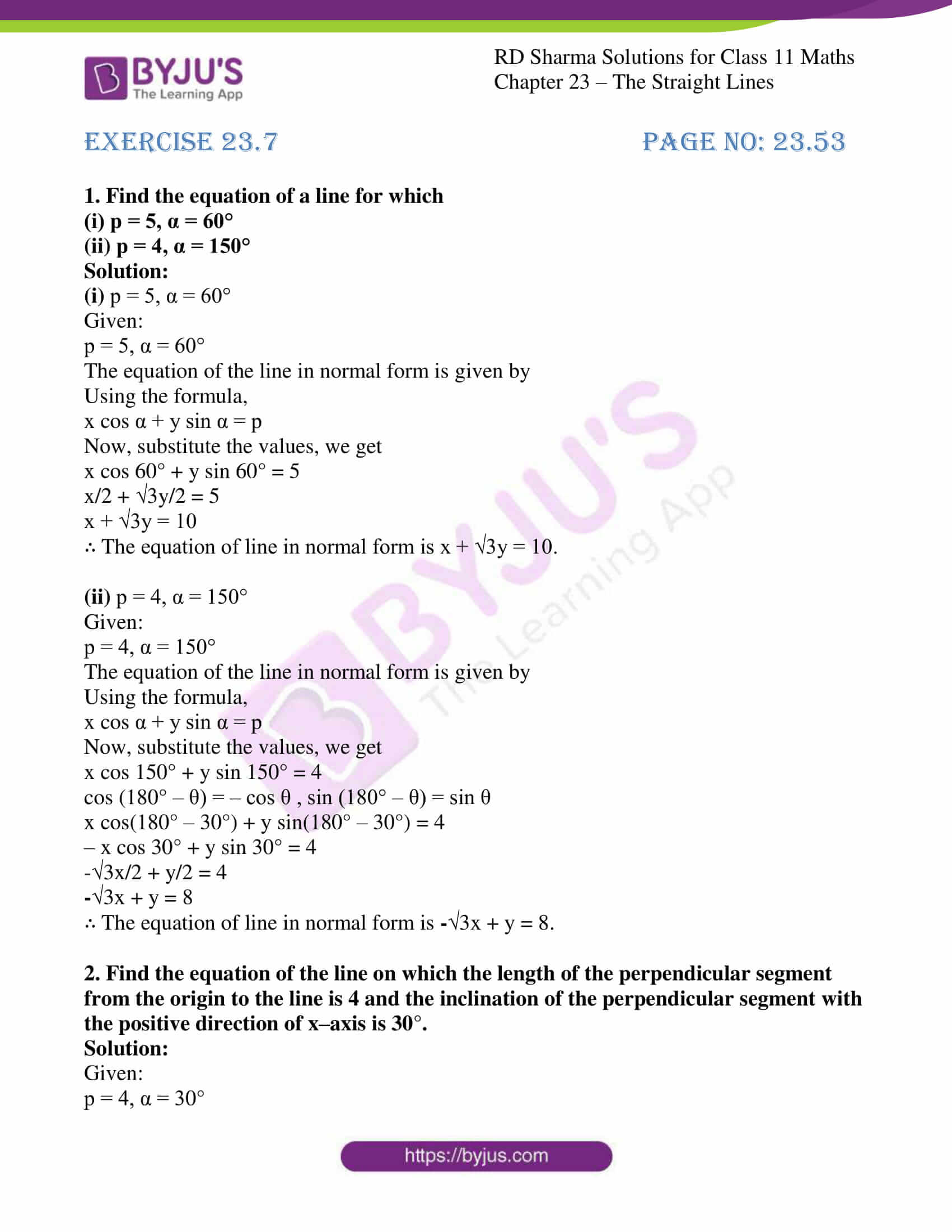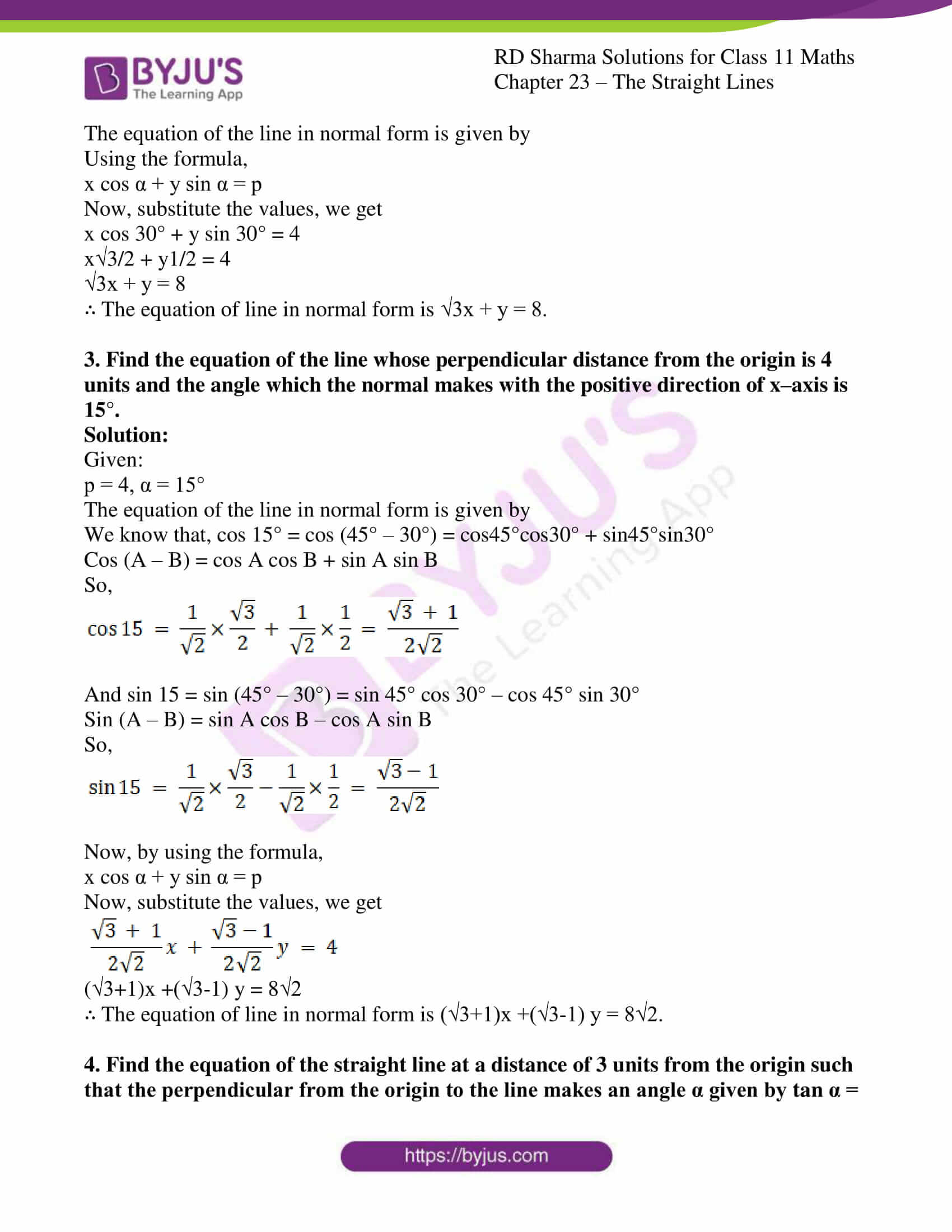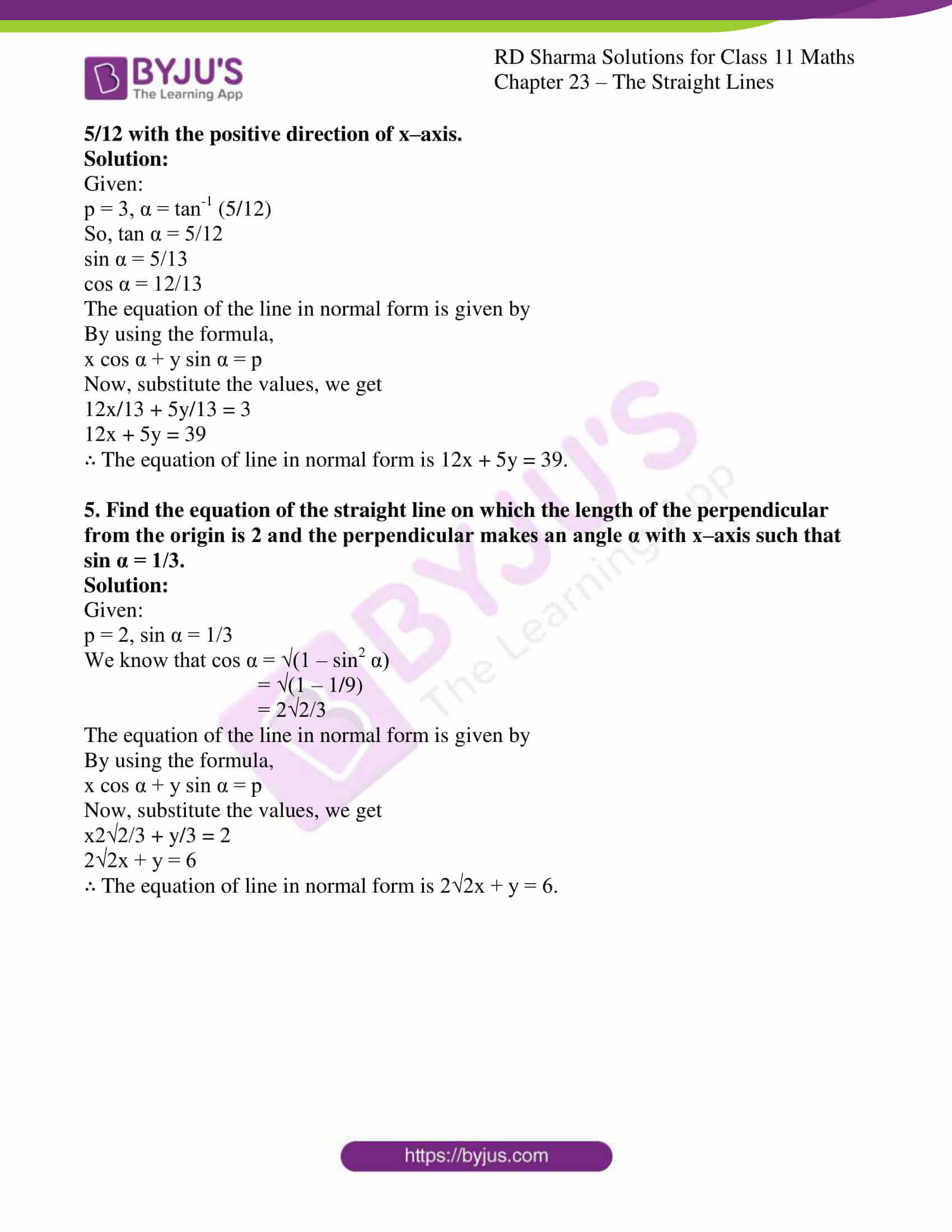### Access answers to RD Sharma Solutions for Class 11 Maths Exercise 23.7 Chapter 23 – The Straight Lines

#### EXERCISE 23.7 PAGE NO: 23.53

1. Find the equation of a line for which

(i) p = 5, α = 60°

(ii) p = 4, α = 150°

Solution:

(i) p = 5, α = 60°

Given:

p = 5, α = 60°

The equation of the line in normal form is given by

Using the formula,

x cos α + y sin α = p

Now, substitute the values, we get

x cos 60° + y sin 60° = 5

x/2 + 3y/2 = 5

x + √3y = 10

∴ The equation of line in normal form is x + √3y = 10.

(ii) p = 4, α = 150°

Given:

p = 4, α = 150°

The equation of the line in normal form is given by

Using the formula,

x cos α + y sin α = p

Now, substitute the values, we get

x cos 150° + y sin 150° = 4

cos (180° – θ) = – cos θ , sin (180° – θ) = sin θ

x cos(180° – 30°) + y sin(180° – 30°) = 4

– x cos 30° + y sin 30° = 4

3x/2 + y/2 = 4

-√3x + y = 8

∴ The equation of line in normal form is -√3x + y = 8.

2. Find the equation of the line on which the length of the perpendicular segment from the origin to the line is 4 and the inclination of the perpendicular segment with the positive direction of x–axis is 30°.

Solution:

Given:

p = 4, α = 30°

The equation of the line in normal form is given by

Using the formula,

x cos α + y sin α = p

Now, substitute the values, we get

x cos 30° + y sin 30° = 4

x3/2 + y1/2 = 4

√3x + y = 8

∴ The equation of line in normal form is √3x + y = 8.

3. Find the equation of the line whose perpendicular distance from the origin is 4 units and the angle which the normal makes with the positive direction of x–axis is 15°.

Solution:

Given:

p = 4, α = 15°

The equation of the line in normal form is given by

We know that, cos 15° = cos (45° – 30°) = cos45°cos30° + sin45°sin30°

Cos (A – B) = cos A cos B + sin A sin B

So,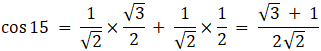And sin 15 = sin (45° – 30°) = sin 45° cos 30° – cos 45° sin 30°

Sin (A – B) = sin A cos B – cos A sin B

So,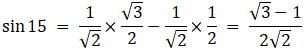Now, by using the formula,

x cos α + y sin α = p

Now, substitute the values, we get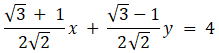(√3+1)x +(√3-1) y = 8√2

∴ The equation of line in normal form is (√3+1)x +(√3-1) y = 8√2.

4. Find the equation of the straight line at a distance of 3 units from the origin such that the perpendicular from the origin to the line makes an angle α given by tan α = 5/12 with the positive direction of x–axis.

Solution:

Given:

p = 3, α = tan-1 (5/12)

So, tan α = 5/12

sin α = 5/13

cos α = 12/13

The equation of the line in normal form is given by

By using the formula,

x cos α + y sin α = p

Now, substitute the values, we get

12x/13 + 5y/13 = 3

12x + 5y = 39

∴ The equation of line in normal form is 12x + 5y = 39.

5. Find the equation of the straight line on which the length of the perpendicular from the origin is 2 and the perpendicular makes an angle α with x–axis such that sin α = 1/3.

Solution:

Given:

p = 2, sin α = 1/3

We know that cos α = √(1 – sin2 α)

= √(1 – 1/9)

= 2√2/3

The equation of the line in normal form is given by

By using the formula,

x cos α + y sin α = p

Now, substitute the values, we get

x2√2/3 + y/3 = 2

2√2x + y = 6

∴ The equation of line in normal form is 2√2x + y = 6.